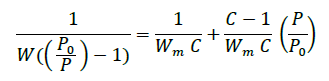# How to Appropriately Characterize the BET Area of a Microporous Material

## Microporous materials are defined as materials containing pores which have diameters less than 2 nm. An important characteristic of all porous materials is the materials surface area, commonly calculated utilizing the BET equation. However for a microporous material, the BET equation in its classical sense provides erroneous results. This report discusses its importance and the appropriate measures to accurately calculate the BET area of microporous materials.

The Brunauer-Emmett-Teller (BET) method is the methodmost widely used to evaluate the surface area of porousmaterial. The BET equation is given by the formula:Where W is the weight of gas adsorbed at a relative pressure of P/P0, and Wm is the weight of the adsorbate constituting a monolayer of surface coverage. The term C, the BET C constant, is related to the energy of adsorption in the first adsorbed layer and consequently its value is an indication of the magnitude of the adsorbent/adsorbate interactions.

The BET equation is applicable for surface area analysis of nonporous, macro-, and mesoporous materials (with sufficiently large pores) and is applied in the relative pressure range P/P0 from 0.05 – 0.3. However, this methodis in a strict sense not applicable in the case of microporous adsorbents – a critical appraisal of the BET method is given in various textbooks on porosity measurement [1-4]. The BET method is based on the assumption that a monolayer, single layer of adsorbate, is formed on the pore walls. In materials possessing micropores, it is difficult to differentiate between the monolayer formation process and the micropore filling process that usually occurs at P/P0 below 0.15. The application of the BET method is also problematic for estimating the specific surface area of mesoporous molecular sieves of pore widths less than ~4 nm because pore condensation is observed at pressures very close to the pressure range where mono layer-multi-layer formation occurs on the pore walls. This may lead to a significant overestimation of the monolayer capacity.

For these reasons, the surface area obtained using the BET equation for microporous materials should be considered a “characteristic” or “equivalent” surface area, or it should just be called BET area. In this case it is, of course, obligatory to report the range of linearity for the BET plot. If the classical BET range (P/P0 - 0.05 to 0.3) is applied to a microporous material isotherm, a non-linear plot with an unacceptable correlation coefficient as well as a negative y-intercept and negative C constant will result - an unphysical result.